# Parallel Self-Tuning Spectral Clustering on Apache Spark

Disclaimer: This story is a short casual version of my master thesis.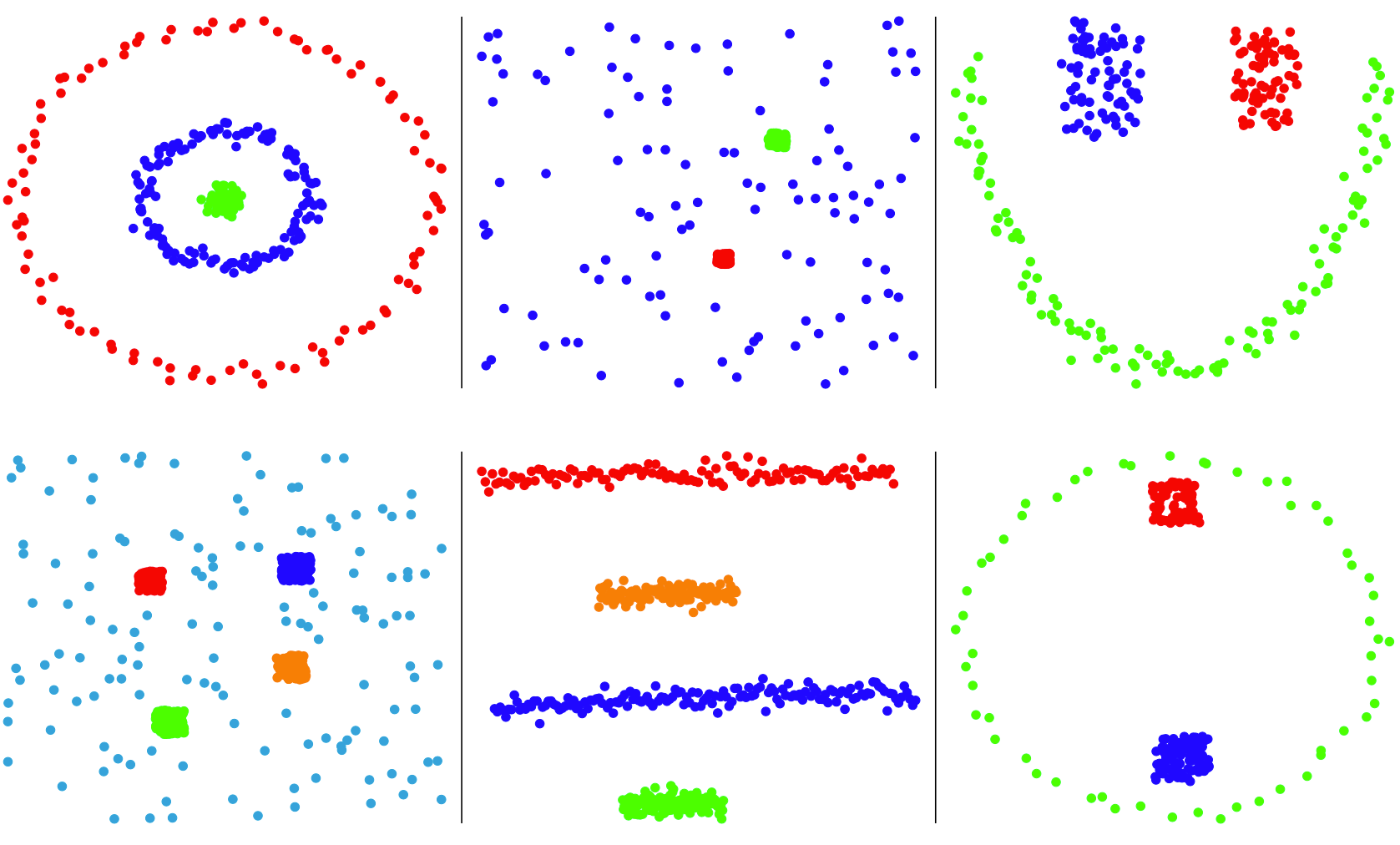Clustering results of the sequential version of the algorithm on simple datasets with maxPossibleGroups = 6.

# Introduction

• Self-Tuning Spectral Clustering (STSC), an algorithm created in 2005 by Lihi Zelnik-Manor and Pietro Perona.
• Parallelization using Apache Spark, an engine designed to process data faster.

Clustering is a common technique for data analysis used to group data objects into clusters, it is the core of this paper. The fundamentals required to understand clustering, spectral clustering, and STSC will be explained in the corresponding part of this story.

# Motivation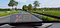A BMW Head-Up Display

Car manufacturers are implementing technologies into their new models to improve drivers’ safety. For example, some of them add cameras to take pictures of the road signs and understand the environment surrounding the vehicle.

Now let us imagine one small addition: instead of keeping this information in the car, why not send it to a server where it can be stored, processed, and then used by all the cars of the manufacturer?

This improvement is a real need and it creates new challenges. Here is an example of two road signs at the exit of a small town:

On the left are the actual positions of the two road signs and on the right the data concerning this exit stored on the server of the car manufacturer. Every time a car takes and processes a picture of a road sign the information is sent thus one road sign corresponds to many observations.

This is why we need to cluster, to group all the observations and be able to find the most likely position and information about each road sign, in the entire Europe.

# Problem

This is a big number, each road sign being represented by many observations and data being added regularly. How do we cluster all these points in order to create a real-time map of Europe?

We need an algorithm that:

• works with an unknown number of clusters, as road signs are added and removed every day,
• recognizes groups of different densities, as a road sign on the side of a highway will be represented by more observation than one in the middle of the countryside,
• can process a high number of observations.

One possible solution is to parallelize the self-tuning spectral clustering algorithm, the subject of this thesis. But how do we parallelize an algorithm that is sequential? By taking advantage of the use case previously defined.

If you are analyzing a road sign in Berlin, you do not care about the road signs in Paris.

The fact that the dataset we wish to analyze is not composed of a few big clusters but a multitude of small ones allows us to cut the dataset.

To do that, we use a kd-tree data structure and an algorithm that creates tiles that contain the same number of observations.

These tiles are then given to different machines and processed in parallel. This solution presents challenges, such as what to do if a cut (a red line on the map) divides a cluster. Solutions have been found and will be described in the “Approach and evaluation” part.

# Fundamentals

## Clustering

The centroid model is relatively simple, it defines each cluster as a group that has a central vector. The most famous algorithm that uses this model is k-means where the clustering is done in three steps: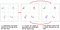The steps 2 and 3 are repeated until there is convergence.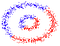A badly clustered dataset using k-means.

This model is straightforward and the computation is fast but the result can change depending on where are the initial means.

Another problem with that model is that it does not work with clusters that are not well separated circular shapes (such as in the example).

Another clustering model is the distribution model, viewing each cluster as a group of objects that belong to the same distribution (e.g. a Normal/Gaussian distribution). If the dataset cannot be represented by a distribution, it will simply not work.

The Expectation Maximization (EM) algorithm follows this model, it is composed of three steps:

1. k randomly placed Gaussians distributions are created in the dataset.
2. Expectation step: the likelihood for every observation to be in each dataset is computed.
3. Maximization step: the mean of each cluster that uses its belonging observations is computed.

The steps 2 and 3 are repeated until convergence, like in k-means (which explains why the EM algorithm is seen as a generalization of the centroid-based algorithm). The probability for each observation to be in every cluster is computed thus the clustering algorithm is defined as soft.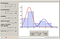A dataset that contains two Gaussian distributions and would be perfectly clustered by the EM algorithm.

The last major clustering model is the density model, it is a really powerful model that works with any cluster’s shapes and even noisy data around the clusters.

This density model requires as inputs the dataset, a maximum distance between two observations in a group and a minimum number of observations required to form a cluster.

As its name suggests, the density is extremely important in this model thus some algorithms such as DBSCAN do not work if clusters do not have the same density. Some variants that use the same model solve this problem, such as OPTICS.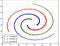A dataset clustered using DBSCAN.

## Spectral clustering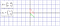An identity matrix A and a vector x1.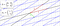A second matrix seen as a linear transformation and the same vector x1 which keeps the same direction.

From the two images above, we can see that the matrix changes a lot but that x1 keeps the same direction. It means that it is an eigenvector of the second matrix A.

Another thing is that x1 is not scaled in the new matrix , which means that the eigenvalue linked to this eigenvector is 1. If x1 had been twice longer in the second image, its corresponding eigenvalue would be 2.

So this is the key to spectral clustering but one question remains: how do we find a matrix from observations?

Spectral clustering are always divided in these four steps:

1. Create an affinity matrix from the dataset that represents the connection between each observation. This matrix is symmetric.
2. Create a Laplacian matrix from the affinity matrix by transforming it (like computing the sum of the affinity matrix columns to create a diagonal matrix and then multiplying it with the affinity matrix).
3. Compute the first k eigenvectors of the Laplacian matrix and create a new matrix where they are columns. The first column is the biggest eigenvector, the second column is the second biggest one and so on.
4. Cluster the rows of the matrix using an algorithm such as k-means, the results are then applied to the original dataset. It means that if the first and third columns of the matrix belongs to the cluster B then the first and third observations in the original dataset are in the same cluster.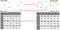The standard steps of spectral clustering algorithms.

Spectral clustering algorithms also work with clusters of many shapes and background noise. The computation of the biggest eigenvectors takes quite some time thus it is an algorithm we only use in tricky cases, not for datasets where k-means would also work well.

## Self-Tuning Spectral Clustering (STSC)

The local scale uses the position of the kth closest observation of each observation and impacts the weight of the affinity between each observation depending on that.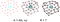In the original paper, k = 7 is said to bring the best result.

The second point, the most important for this thesis, is the fact that STSC does not take a number k of eigenvectors from the Laplacian. Instead, it will be able to find the most likely number of clusters in a dataset from 2 to k.

How does this work? Using the Laplacian matrix, the algorithm first takes the two eigenvectors and applies a Givens rotation to reduce a value called the cost (which computes the difference between the biggest value of each row and the others).

Once the cost is minimal, it is saved and the third biggest eigenvector is added as the third column of the rotated matrix. We repeat the same minimization of the matrix → comparison of the costs → addition of an eigenvector process up to k.

If the new cost is better than any previous one, we save the rotated matrix. Once we have computed the cost up to k, we use this matrix and cluster its rows as in any spectral clustering algorithm.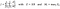The computation of the cost, Z is the rotated matrix and Mi the maximum value in its row i.

By not asking for a specific number k but only a maximum possible number of groups of clusters, the algorithm is defined as self-tuned. The only arguments required for running the algorithm are the dataset and this number.

# Approach and evaluation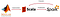The main steps of my master thesis.

There were a number of quirks in the original implementation:

• It did not strictly follow the original paper, many values explicitly described in the paper were changed and some things were different with no explicit reasons (e.g. using a value called the quality instead of the cost to compare the quality of the rotations).
• It has been developed in MATLAB thus it requires a licence in order to run the code.
• It was not developed as a library, there were only two examples and extremely few comments along the code.

It took way more time to transform this MATLAB code into Scala than I imagined. The new implementation:

• follows the original paper without exception. A new approach to find the best rotation of a matrix has also been developed, faster and offering a better minimalization than the two implemented in the original code,
• is usable (and has been used) as a Java library,
• has unit tests, a JavaDoc and also inline comments. The entire sequential algorithm is contained in one file.

To compete with MATLAB, I have extensively used a linear algebra library called Breeze. The code is thus concentrated on the computation of the algorithm, not on how to create a matrix structure.

To compare the old and new implementations, I have checked the results on the datasets that were given with the original code. The results can be seen in the first image of this article and they are the same for the two implementations.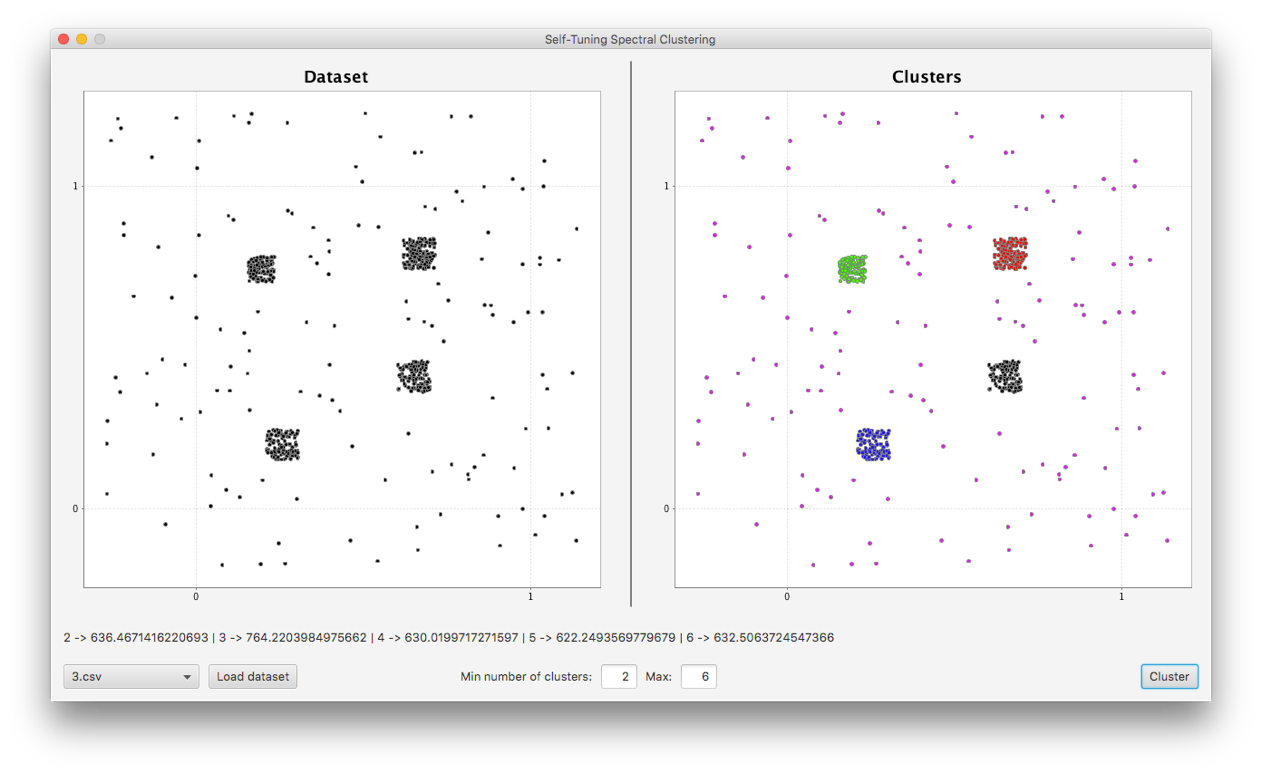To test the sequential algorithms, I have used my code as a library and created different graphical user interfaces.

Once the sequential algorithm was done, I started the parallel implementation. The main question to solve was how to handle a situation where a tile cuts a cluster and thus does not contain all its observations. The solution introduced for this thesis is called the border width.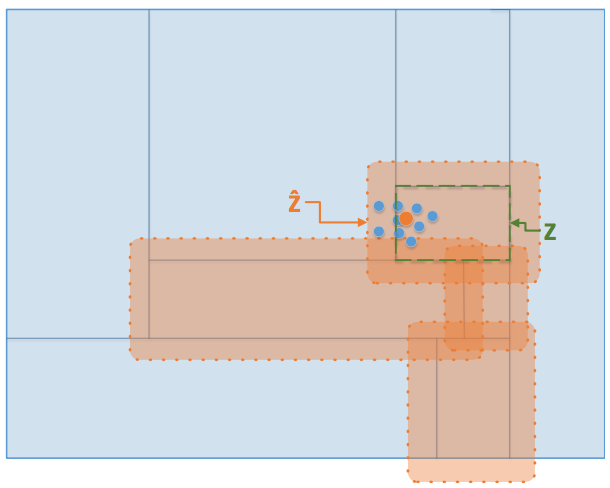The tile Z and its border width Ẑ.

The border width is a value given by the user when clustering a dataset in parallel.

During the step where we get all the observations for a tile before sending them to a machine, we take all the ones strictly in the tile but also the ones around the tile, included in the border width.

This means that an observation can be in multiple tiles.

So what do we do in order not to corrupt the clustering by having the same observation in two different clusters? We add one more step after clustering each tile in parallel.

We compute the center of each cluster and, if it is not strictly in the tile, we remove it and all its observations from the tile. This step ensures us that an observation is not present multiple times in the final output.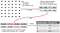The main steps of the parallel algorithm.

The last step was the comparison of the two algorithms: sequential v. parallel. The tests have been done on a Google Cloud instance with 4 CPUs and 10 GB of RAM. For the test of the parallel algorithm, two Spark workers were configured to have 1 CPU and 4GB of RAM each.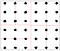The dataset with the cuts of the kd-tree displayed in red.

The dataset used for the test was composed of 36 well separated clusters each following a multi-variate Gaussian distribution.

The sequential implementation was given an input maxPossibleGroups = 40 and the parallel implementation an input maxPossibleGroupsPerTile = 6.

The kd-tree was created using a function where a maximum number of tiles is given as an input (here 16).

It took 18237s (approximately 5 hours) for the sequential algorithm to find the 36 clusters. It took 0.2s to create the kd-tree, a surprisingly good result even if we need to compute medians in order to know where to cut. It then took 42s to process the dataset in parallel and find the 36 clusters.

The parallel implementation is faster by an order of magnitude. Why? To find an answer, I have applied the sequential algorithm on another dataset composed of 50 clusters of 20 observations and checked the computation time to find the best rotations at each step.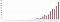Computation time in seconds to find the best rotation for the matrix composed of X-1 already rotated eigenvectors and the Xth eigenvector.

This increase is due to the computation of the best rotations. Let us take the simplest case: the first step with 2 eigenvectors. We take the two biggest ones of the Laplacian and we want to rotate them in order to minimize the cost function.

For a matrix composed of two columns, we only have (N * (N-1)) / 2 = 1 θ to compute. It means that we only have one value θ that we can change in order to rotate the matrix and thus modify the cost J.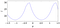The modification of the cost depending on θ for a matrix of two columns.

Using derivatives, it is simple to find the best θ for having a minimal cost in this case. But how many θ do we have to find for a matrix composed of 20 columns? (20 * 19) / 2 = 190 θ, each depending on the others.

The time complexity of the algorithm is thus O(X²), which explains why cutting the dataset and use a smaller value maxPossibleGroups is way faster. In fact, just cutting the dataset and processing it sequentially (thus, without Apache Spark) would still be faster than the original algorithm!

# Conclusion

What has been found? One thing that has not been discussed but that you may have guessed when reading the computation of the cost: the algorithm does not work if there is only 1 cluster in the dataset. This is really bad for a clustering algorithm and the fact that it was not written in the original paper is quite astonishing.

The other problems are the computation time with many clusters and the fact that the quality of the algorithm seemed to decrease when computing datasets composed of many clusters. The costs seemed to reach a plateau around the most likely number of clusters in the dataset and sometimes induce the algorithm in error.

What could be done? The algorithm has only been tested in 1 and 2 dimensions during this thesis, it is said in the original paper that it works great in more dimensions but there is no proof of that thus it would be great to work on this matter.

Last but not least, there is a chicken and egg problem in the parallel algorithm. The computation is defined as self-tuned but we now need a value called the border width that should be half the length of the biggest cluster in each dimension in order to have an optimal result/computation time.

This value cannot currently be obtained without having a look at the dataset, finding a way to compute it automatically would be a great achievement in order to keep the algorithm self-tuned.

The parallel algorithm developed is a solution for the stated use case of a dataset composed of a lot of clusters. Even if this implementation only works for a few datasets containing small clusters, the development of a sequential version that is actually usable and testable gives the opportunity to try STSC in many cases.

By offering a solution for people to try the algorithm instead of limiting themselves to the examples given in the paper, new qualities and drawbacks can be (and have been) found.

# Learn more

Senior software engineer working remotely at D2iQ (formerly Mesosphere).

## More from Armand Grillet

Senior software engineer working remotely at D2iQ (formerly Mesosphere).